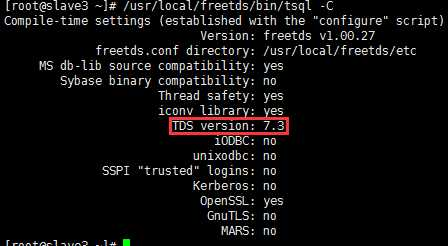# sql 普通行列转换_MsSql

select fangabh,listagg(duifbsh,'/')within group(order by fangabh desc)from tablegroup by fangabh如果是oracle的话，也可以wmsys.wm_concat，如下select fangabh,wmsys.wm_concat(duifbsh)duifbsh,count(duifbsh)as tt from tablegroup by fangabhorder by tt descwww.zgxue.com防采集请勿采集本网。-mssql: with tmp(col)as (select '1111' union all select '2222' union all select '3333' union all select '4444'), tmp1(col,col_new,level)as (select col,cast(SUBSTRING(col,1,1)as

sqlserver2005中可以用 Pivot UnPivot 来实现。sqlserver2000的话，行数 和列数在数据库中是可变的，恐怕很难实现。想想存储过程+临时表吧。

---- ---- ---- ----

-------------------

*/

create table tb(姓名 varchar(10) , 课程 varchar(10) , 分数 int)

insert into tb values('张三' , '语文' , 74)

insert into tb values('张三' , '数学' , 83)

insert into tb values('张三' , '物理' , 93)

insert into tb values('李四' , '语文' , 74)

insert into tb values('李四' , '数学' , 84)

insert into tb values('李四' , '物理' , 94)

go

--SQL SERVER 2000 静态SQL,指课程只有语文、数学、物理这三门课程。(以下同)

select 姓名 as 姓名 ,

max(case 课程 when '语文' then 分数 else 0 end) 语文,

max(case 课程 when '数学' then 分数 else 0 end) 数学,

max(case 课程 when '物理' then 分数 else 0 end) 物理

from tb

group by 姓名

--SQL SERVER 2000 动态SQL,指课程不止语文、数学、物理这三门课程。(以下同)

declare @sql varchar(8000)

set @sql = 'select 姓名 '

select @sql = @sql + ' , max(case 课程 when ''' + 课程 + ''' then 分数 else 0 end) [' + 课程 + ']'

from (select distinct 课程 from tb) as a

set @sql = @sql + ' from tb group by 姓名'

exec(@sql)

--SQL SERVER 2005 静态SQL。

select * from (select * from tb) a pivot (max(分数) for 课程 in (语文,数学,物理)) b

--SQL SERVER 2005 动态SQL。

declare @sql varchar(8000)

select @sql = isnull(@sql + '],[' , '') + 课程 from tb group by 课程

set @sql = '[' + @sql + ']'

exec ('select * from (select * from tb) a pivot (max(分数) for 课程 in (' + @sql + ')) b')

---------------------------------

/*

---- ---- ---- ---- ------ ----

*/

--SQL SERVER 2000 静态SQL。

select 姓名 姓名,

max(case 课程 when '语文' then 分数 else 0 end) 语文,

max(case 课程 when '数学' then 分数 else 0 end) 数学,

max(case 课程 when '物理' then 分数 else 0 end) 物理,

cast(avg(分数*1.0) as decimal(18,2)) 平均分,

sum(分数) 总分

from tb

group by 姓名

--SQL SERVER 2000 动态SQL。

declare @sql varchar(8000)

set @sql = 'select 姓名 '

select @sql = @sql + ' , max(case 课程 when ''' + 课程 + ''' then 分数 else 0 end) [' + 课程 + ']'

from (select distinct 课程 from tb) as a

set @sql = @sql + ' , cast(avg(分数*1.0) as decimal(18,2)) 平均分 , sum(分数) 总分 from tb group by 姓名'

exec(@sql)

--SQL SERVER 2005 静态SQL。

select m.* , n.平均分 , n.总分 from

(select * from (select * from tb) a pivot (max(分数) for 课程 in (语文,数学,物理)) b) m,

(select 姓名 , cast(avg(分数*1.0) as decimal(18,2)) 平均分 , sum(分数) 总分 from tb group by 姓名) n

where m.姓名 = n.姓名

--SQL SERVER 2005 动态SQL。

declare @sql varchar(8000)

select @sql = isnull(@sql + ',' , '') + 课程 from tb group by 课程

exec ('select m.* , n.平均分 , n.总分 from

(select * from (select * from tb) a pivot (max(分数) for 课程 in (' + @sql + ')) b) m ,

(select 姓名 , cast(avg(分数*1.0) as decimal(18,2)) 平均分 , sum(分数) 总分 from tb group by 姓名) n

where m.姓名 = n.姓名')

drop table tb

------------------

------------------

/*---- ---- ----

sqlserver2005中可以用 Pivot UnPivot 来实现。sqlserver2000的话，行数 和列数在数据库中是可变的，恐怕很难实现。想想存储过程+临时表吧。

-mssql: with tmp(col)as (select '1111' union all select '2222' union all select '3333' union all select '4444'), tmp1(col,col_new,level)as (select col,cast(SUBSTRING(col,1,1)as

--------------

*/

create table tb(姓名 varchar(10) , 语文 int , 数学 int , 物理 int)

insert into tb values('张三',74,83,93)

insert into tb values('李四',74,84,94)

go

--SQL SERVER 2000 静态SQL。

select * from

(

select 姓名 , 课程 = '语文' , 分数 = 语文 from tb

union all

select 姓名 , 课程 = '数学' , 分数 = 数学 from tb

union all

select 姓名 , 课程 = '物理' , 分数 = 物理 from tb

) t

order by 姓名 , case 课程 when '语文' then 1 when '数学' then 2 when '物理' then 3 end

--SQL SERVER 2000 动态SQL。

--调用系统表动态生态。

declare @sql varchar(8000)

select @sql = isnull(@sql + ' union all ' , '' ) + ' select 姓名 , [课程] = ' + quotename(Name , '''') + ' , [分数] = ' + quotename(Name) + ' from tb'

from syscolumns

where name! = N'姓名' and ID = object_id('tb') --表名tb，不包含列名为姓名的其它列

order by colid asc

exec(@sql + ' order by 姓名 ')

--SQL SERVER 2005 动态SQL。

select 姓名 , 课程 , 分数 from tb unpivot (分数 for 课程 in([语文] , [数学] , [物理])) t

--SQL SERVER 2005 动态SQL，同SQL SERVER 2000 动态SQL。

--------------------

/*---- ------ ------

------------------

*/

select * from

(

select 姓名 as 姓名 , 课程 = '语文' , 分数 = 语文 from tb

union all

select 姓名 as 姓名 , 课程 = '数学' , 分数 = 数学 from tb

union all

select 姓名 as 姓名 , 课程 = '物理' , 分数 = 物理 from tb

union all

select 姓名 as 姓名 , 课程 = '平均分' , 分数 = cast((语文 + 数学 + 物理)*1.0/3 as decimal(18,2)) from tb

union all

select 姓名 as 姓名 , 课程 = '总分' , 分数 = 语文 + 数学 + 物理 from tb

) t

order by 姓名 , case 课程 when '语文' then 1 when '数学' then 2 when '物理' then 3 when '平均分' then 4 when '总分' then 5 end

drop table tb

SELECTMAX(CASE WHEN SetupID=1 THEN SetupName ELSE NULL)AS,MAX(CASE WHEN SetupID=2 THEN SetupName ELSE NULL)AS,MAX(CASE WHEN SetupID=3 THEN SetupName ELSE NULL)AS,MAX(CASE WHEN SetupID=4 THEN SetupName ELSE NULL)ASFROM table内容来自www.zgxue.com请勿采集。

• 本文相关：
• 一个简单的sql 行列转换语句
• sqlserver2005 行列转换实现方法
• c#中datatable实现行列转换的方法
• 浅析sql语句行列转换的两种方法 case...when与pivot函数的应用
• 深入sql中pivot 行列转换详解
• sql实现行列转换方便了我们存储数据和呈现数据
• 玩转-sql2005数据库行列转换
• oracle实现行列转换的方法分析
• js控制网页动态生成任意行列数表格的方法
• 数据库实现行列转换(mysql示例)
• truncate 快速删除表中的所有数据
• sql server安装完成后3个需要立即修改的配置选项
• c#连接数据库及sql2005远程连接的方法
• sql server误区30日谈 第13天 在sql server 2000兼容模式下不能使
• ms sql server 数据库日志压缩方法与代码
• sqlserver实现树形结构递归查询(无限极分类)的方法
• 根据日期知道当天是星期几的手动计算方法
• sql数据库的高级sql注入的一些知识
• sql注入原理简介_动力节点java学院整理
• sql server如何利用开窗函数over()进行分组统计
• SQL 进行行列转换
• sql 如何实现行列转换如图
• 行列转换，求最简单SQL方法，悬赏5分请高手指点！
• Sql怎么实现行列转换
• SQL语句之合并行列转换
• SQL行列转换
• 一个数据行列转换的问是。求SQL 语句。
• sql行列转换
• SQL里行列转换
• SQL行列转换
• 免责声明 - 关于我们 - 联系我们 - 广告联系 - 友情链接 - 帮助中心 - 频道导航